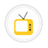you are here->home->Physics->Class 11
Class 11
Class 11Vernier CalipersUse of Vernier Calipers (i)To measure the diameter of a small spherical / cylindrical body. (ii)To measure the length, width and height of the given rectangular block. (iii)To measure the internal diameter and depth of a given beaker/calorimeter and hence find its volume.Inclined PlaneTo find the downward force, along an inclined plane, acting on a roller due to gravitational pull of the earth and study its relationship with the angle of inclination () by plotting graph between force and sin θParallelogram Law of Vectors.To find the weight of a given body using parallelogram law of vectors.Newton's Law of Cooling(Thermal Radiation)To study the relationship between the temperature of a hot body and time by plotting a cooling curve.Resonance ColumnTo find the speed of sound in air at room temperature using a resonance tube by two resonance positions.Young's ModulusDetermine Young's modulus of elasticity of the material of a given wireScrew GaugeUse of screw gauge:(i) to measure diameter of a given wire and (ii) to measure thickness of a given sheet.Simple pendulumTo plot L-T and L-T2 graphs using a simple pendulum and thus find the; Effective length of the second's pendulum using the appropriate graph. Acceleration due to gravity.SpherometerTo measure the thickness of a glass strip and to determine the radius of curvature of a given spherical surface.Beam BalanceTo find the mass of the given body by sensibility method, using a beam balance.FrictionTo study the relationship between force of limiting friction and normal reaction and to find the co-efficient of friction between a block and a horizontal surfaceHelical SpringTo find the force constant of a helical spring by plotting a graph between load and extension.Surface TensionTo determine the surface tension of water by capillary rise method.Viscosity of a liquid - Stoke's methodTo determine the coefficient of viscosity of a given viscous liquid by measuring terminal velocity of a given spherical body.Boyle's LawTo study the variation in volume with pressure for a sample of air at constant temperature by plotting graphs between P and V, and between P and 1/v.Sonometer1. To study the relation between frequency and length of a given wire under constant tension using sonometer. To plot a graph between ν and l. 2. To study the relation between length of a given wire and tension for constant frequency using sonometer. To plot a graph between l2 and T.